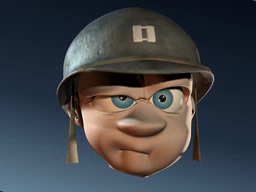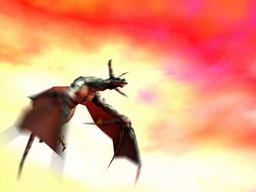# Perceptually Optimized MPEG Compression of Synthetic Video Sequences

Enrico Masala, Davide Quaglia

The performance of the first and the second strategy are tested with four synthetic video sequences created and animated by 3D Studio Max v.5. An automatic object classifier was developed; it consider an object as important if its distance from the point of view is less than one fourth of the farthest object in the scene. The importance of the objects is then used to decide the quantization stepsize (QP) of the corresponding macroblocks. For Strategy 1, we assigned a different QP value to important (QPi) and less important (QPni) macroblocks. For Strategy 2, macroblocks containing the edges of important objects are quantized with a fixed QP value (QPb) which is lower than QPi and QPni.

Frames are encoded by the TM5 MPEG 2 encoder which has been modified to support the proposed techniques. The default GOP structure consists of an I-frame followed by eleven P-frames.

Bitstreams encoded with the proposed techniques are compared with model-unaware bitstreams encoded using fixed values of the quantization stepsize. MPEG bitstreams and sample frames are available below for perceptual evaluation.

## Sequence ComplexFixed QP=31 MPEG file (135975 byte) Sample frame (139769 byte) Fixed QP=13 MPEG file (329247 byte) Sample frame (239142 byte) Fixed QP=5 MPEG file (922369 byte) Sample frame (304033 byte) Strategy 1:  QPi=13,  QPni=31 MPEG file (281812 byte) Sample frame (193335 byte) Strategy 1+2:  QPi=13,  QPni=31,  QPb=5 MPEG file (459257 byte) Sample frame (206310 byte)

## Sequence AnibalFixed QP=31 MPEG file (287815 byte) Sample frame (118418 byte) Fixed QP=13 MPEG file (465944 byte) Sample frame (130725 byte) Fixed QP=5 MPEG file (1112461 byte) Sample frame (141158 byte) Strategy 1:  QPi=13,  QPni=31 MPEG file (466560 byte) Sample frame (130761 byte) Strategy 1+2:  QPi=13,  QPni=31,  QPb=5 MPEG file (697139 byte) Sample frame (133947 byte)

## Sequence DinoFixed QP=31 MPEG file (130373 byte) Sample frame (214095 byte) Fixed QP=13 MPEG file (353345 byte) Sample frame (329565 byte) Fixed QP=5 MPEG file (902743 byte) Sample frame (382775 byte) Strategy 1:  QPi=13,  QPni=31 MPEG file (331237 byte) Sample frame (293890 byte) Strategy 1+2:  QPi=13,  QPni=31,  QPb=5 MPEG file (468725 byte) Sample frame (298812 byte)

## Sequence DragonFixed QP=31 MPEG file (105182 byte) Sample frame (128857 byte) Fixed QP=13 MPEG file (189667 byte) Sample frame (162642 byte) Fixed QP=5 MPEG file (445932 byte) Sample frame (203963 byte) Strategy 1:  QPi=13,  QPni=31 MPEG file (168114 byte) Sample frame (135472 byte) Strategy 1+2:  QPi=13,  QPni=31,  QPb=5 MPEG file (296253 byte) Sample frame (137083 byte)